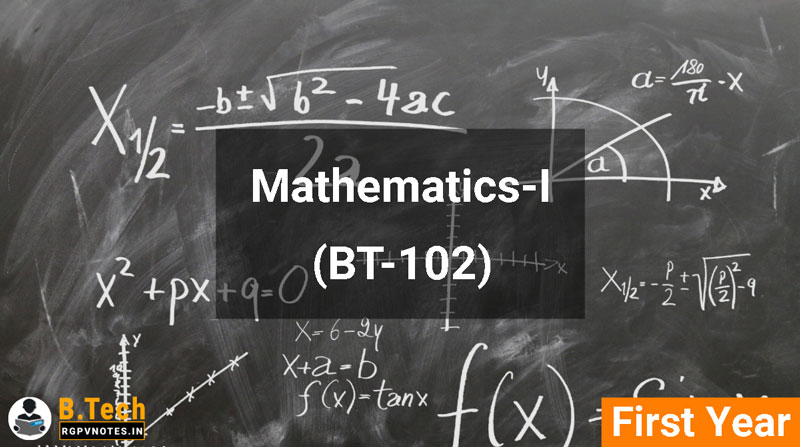Mathematics-I (BT-102) - B Tech RGPV AICTE Flexible Curricula Notes

## Sunday, December 30, 2018

##### Mathematics-I (BT-102)## OBJECTIVES

The objective of this course is to familiarize the prospective engineers with techniques in calculus, multivariate analysis and linear algebra. It aims to equip the students with standard concepts and tools at an intermediate to advanced level that will serve them well towards tackling more advanced level of mathematics and applications that they would find useful in their disciplines. More precisely, the objectives are:
To introduce the idea of applying differential and integral calculus to notions of curvature and to improper integrals. Apart from some applications it gives a basic introduction on Beta and Gamma functions.
To introduce the fallouts of Rolle’s Theorem that is fundamental to application of analysis to Engineering problems.
To develop the tool of power series and Fourier series for learning advanced Engineering Mathematics.
To familiarize the student with functions of several variables that is essential in most branches of engineering.
To develop the essential tool of matrices and linear algebra in a comprehensive manner.

## Syllabus

UNIT 1:
Calculus:
Rolle’s theorem, Mean Value theorems, Expansion of functions by Mc. Laurin’s and Taylor’s for one variable; Taylor’s theorem for function of two variables, Partial Differentiation, Maxima & Minima (two and three variables), Method of Lagranges Multipliers.

UNIT 2:
Calculus:
Definite Integral as a limit of a sum and Its application in summation of series; Beta and Gamma functions and their properties; Applications of definite integrals to evaluate surface areas and volumes of revolutions. Multiple Integral, Change the order of the integration, Applications of multiple integral for calculating area and volumes of the curves.

UNIT 3:
Sequences and series:
Convergence of sequence and series, tests for convergence; Power series, Taylor's series, series for exponential, trigonometric and logarithm functions; Fourier series: Half range sine and cosine series, Parseval’s theorem.

UNIT 4:
Vector Spaces
Vector Space,Vector Sub Space, Linear Combination of Vectors,Linearly Dependent, Linearly Independent, Basis of a Vector Space,Linear Transformations.

UNIT 5:
Matrices
Rank of a Matrix, Solution of Simultaneous Linear Equations by Elementary Transformation, Consistency of Equation, Eigen Values and Eigen Vectors, Diagonalization of Matrices, Cayley-Hamilton theorem and its applications to find inverse.

• Unit 1
• Unit 2
• Unit 3
• Unit 4
• Unit 5

## References

1. G.B. Thomas and R.L. Finney, Calculus and Analytic geometry, 9th Edition,Pearson, Reprint, 2002.
2. Erwin kreyszig, Advanced Engineering Mathematics, 9th Edition, John Wiley & Sons, 2006.
3. Veerarajan T., Engineering Mathematics for first year, Tata McGraw-Hill, New Delhi, 2008.
4. Ramana B.V., Higher Engineering Mathematics, Tata McGraw Hill New Delhi, 11thReprint, 2010.
5. D. Poole, Linear Algebra: A Modern Introduction, 2nd Edition, Brooks/Cole, 2005.
6. N.P. Bali and Manish Goyal, A text book of Engineering Mathematics, Laxmi Publications, Reprint, 2008.
7. B.S. Grewal, Higher Engineering Mathematics, Khanna Publishers, 36th Edition, 2010.

## You May Also Like

#### Services

###### COMPLETELY FREE !!!

Yup, everything is free....

###### NO REGISTRATION REQUIRED

User doesn't have to register for accessing the files, all the files are free & universally accessible without any condition or restriction.

###### RESPONSIVE DESIGN & USER-FRIENDLY

Our webpages are responsive & user-friendly, which means it will automatically adjust according to your device screen size and you will find stuff without ant hustle.

All the files are uploaded on our super-fast servers so that they can be easily downloaded with high speed.

###### NEW PROJECTS

For providing a better experience to our users we are developing our Android application, the application will have a lot of awesome features so stay tuned ;).

###### AWESOME SUPPORT TEAM

Our AI-powered Chatbots are always here to help you so, feel free to ask any question or report if you face any problem. Our team also monitors all chatbots traffic & they will contact you if chatbot fails to help.